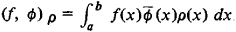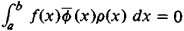# Orthogonality

(redirected from Orthogonak)
Also found in: Dictionary, Thesaurus.

## orthogonality

[ȯr‚thäg·ə′nal·əd·ē]
(mathematics)
Two geometric objects have this property if they are perpendicular.

## Orthogonality

a generalization and often a synonym of the concept of perpendicularity. If two vectors in three-dimensional space are perpendicular, their scalar product is equal to zero. This fact permits us to generalize the concept of perpendicularity by extending it to vectors in any linear space, where a scalar product is defined with the usual properties. Thus two vectors are said to be orthogonal if their scalar product is equal to zero. In particular, let us define the scalar product in the space of complex-valued functions on the interval [a, b] by the formulawhere ρ(x) ≥ 0. Then, if (f, ϕ)ρ = 0, that is,f(x) and ϕ(x) are said to be orthogonal with respect to the weight function ρ(x). Two linear subspaces are termed orthogonal if every vector in one of them is orthogonal to every vector in the other. This concept generalizes the concept of the perpendicularity of two lines or of a line and a plane in three-dimensional space but not the concept of the perpendicularity of two planes. Curves that intersect at right angles, as measured by the angle between the tangents at the point of intersection, are called orthogonal curves.

Site: Follow: Share:
Open / Close